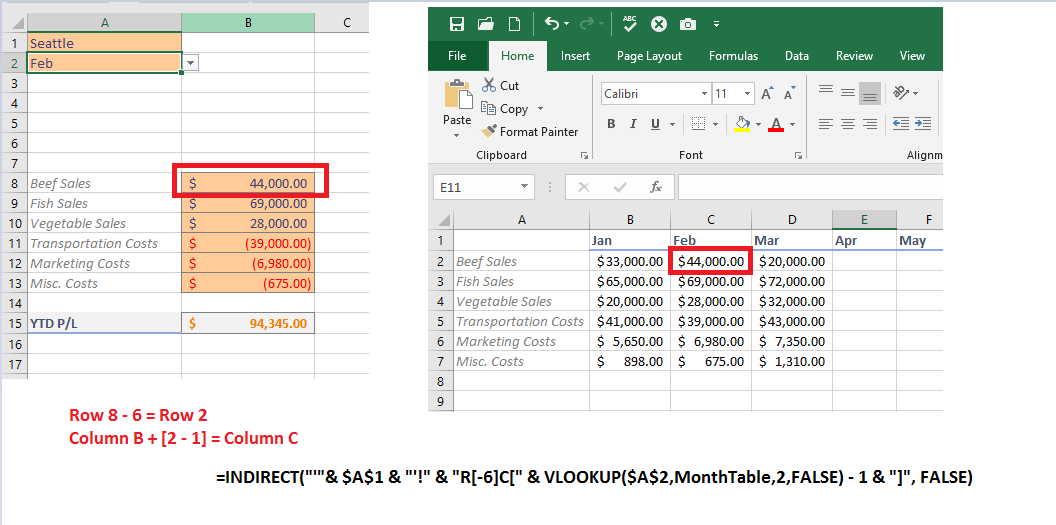# Excel Profit and Loss – INDIRECT and VLOOKUP

You can build an Excel profit and loss spreadsheet using the INDIRECT and VLOOKUP functions. Together, these functions enable you to analyze profit and expense data for your projects.

I’ll demonstrate how these functions can be combined to help you break analysis up by month and a specific region / city. This is a somewhat advanced topic where I’ll assume you understand the Microsoft Excel syntax for worksheet, cell, and range references. As you read through, imagine how you can use this configuration for anything ranging from the food-and-beverage industry to software development project P/L analysis.

## Excel Profit and Loss Layout and Function

Your workbook and data need to be structured in a specific-and-consistent way in order to use the INDIRECT and VLOOKUP functions together for profit and loss analysis as demonstrated in this post. I’ll be looking at the overall P/L for various food items that are distributed and sold throughout the United States. That is, sales versus the cost of transportation, marketing, and other factors.

Review the video below. You should take away requirements that lead into the upcoming explanation of how to use INDIRECT and VLOOKUP to build this spreadsheet:

• All data is structured exactly the same for sales and expenses in each of the per-city worksheets. EXACTLY the same; this is important because the references demonstrated later depend on specific cell locations.
• I’ve created Cities and Months tables with named tables and named ranges. These are used to inform Data Validation settings for the list drop-downs seen on the “Analysis” worksheet.

Not so important, but worth calling out: I don’t have calculations for Apr – Dec yet as I’m posting this on 4/9/2019. Though this is fictitious to some degree, it’s important to highlight that everything functions even when an entire year’s data is not yet available.

Let’s now review how INDIRECT and VLOOKUP were combined to build the main “Analysis” worksheet.

# INDIRECT and VLOOKUP

The formula that powers B8 through B13:

`=INDIRECT("'"& \$A\$1 & "'!" & "R[-6]C[" & VLOOKUP(\$A\$2,MonthTable,2,FALSE) - 1 & "]", FALSE)`
• The INDIRECT function interpolates the value specified in argument 1 as a literal string reference to some cell in the document.
• The second argument, as FALSE, is setting an option that that the syntax in argument one will specify an exact Row and Cell Reference offset from the existing row and cell.

Let’s break this down. A user chooses “Detroit” from A1 and then “Jan” from A2.

That get converted into the string ‘Detroit’!R[-6]C

Written out explicitly: go to the Detroit tab, grab the value that is 6 rows above the current row and 0 columns to the right of the current column.

If the user selects “Detroit” from A1 and then “Feb” from “A2”, that’s interpreted as: go to the Detroit tab, pull the value that is 6 rows above the current row and 1 column to the right of the current column.

The same formula, identical, is copied into B8:B13. The only change is that I add a “* -1” to B11:B13 so that they are negative values. Then, I use the Microsoft Excel SUM function

`=SUM(B8:B13)`

to calculate the total in B15.

So, for Seattle in February:And, when you copy the formula down, you don’t really need to worry about absolute references because this formula is relative by design. INDIRECT works by calculating offsets from the current row and column.

## Excel P&L Summary

As you saw from the first video demonstration, everything adjusts accordingly as you change the city and month. The reference to the worksheet is driven by the value selected in A1 (City), and the offset reference for column is set by the VLOOKUP in Months!MonthTable[MonthOffset].

1.•